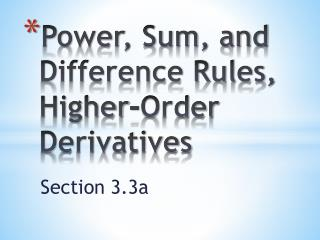DownloadDownload PresentationPower, Sum, and Difference Rules, Higher-Order Derivatives

# Power, Sum, and Difference Rules, Higher-Order Derivatives

Télécharger la présentation## Power, Sum, and Difference Rules, Higher-Order Derivatives

- - - - - - - - - - - - - - - - - - - - - - - - - - - E N D - - - - - - - - - - - - - - - - - - - - - - - - - - -
##### Presentation Transcript

1. The “Do Now” Find the derivative of Does this make sense graphically???

2. The “Do Now” Find the derivative of Let’s see some patterns so we can generalize some easier rules for finding these derivatives…

3. Suppose is a function with a constant value c. Then Rule 1: Derivative of a Constant Function If is the function with the constant value c, then

4. If , then the difference quotient for is: Recall the algebraic identity: Here, we will let and : What happens when we let h 0?

5. Rule 2: Power Rule for Integer Powers of x If is an integer, then n The Power Rule says: To differentiate x , multiply by n and subtract 1 from the exponent.

6. Suppose we have a function comprised of a differentiable function of x multiplied by a constant: Then Rule 3: The Constant Multiple Rule If is a differentiable function of x and c is a constant, then The constant “goes along for the ride”

7. Returning to the “Do Now” Find the derivative of Find the derivative of

8. What if we have a function comprised of a sum of other differentiable functions? The derivative:

9. Rule 4: The Sum and Difference Rule If u and v are differentiable functions of x, then their sum and difference are differentiable at every point where u and v are differentiable. At such points, The derivative of a sum (or difference) is the sum (or difference) of the derivatives

10. Guided Practice Find if Take each term separately:

11. Guided Practice Find if With no negative exponents:

12. Second and Higher Order Derivatives The derivative is called the first derivative of y with respect to x. The first derivative may itself be a differentiable function of x. If so, its derivative is called the second derivative of y with respect to x. If (“y double-prime”) is differentiable, its derivative is called the third derivative of y with respect to x. The pattern continues…

13. Second and Higher Order Derivatives However, the multiple-prime notation gets too cumbersome after three primes. We use to denote the n-th derivative of y with respect to x. (We also use .) Note: Do not confuse with the n-th power of y, which is

14. Guided Practice Find the first four derivatives of . First derivative: Second derivative: Third derivative: Fourth derivative:

15. Guided Practice Does the curve have any horizontal tangents? If so, where? If there are any horizontal tangents, they occur where the slope dy/dx is zero… Solve the equation dy/dx = 0 for x: How can we support our answer graphically?